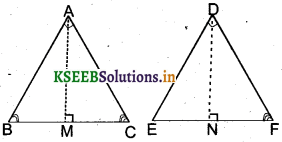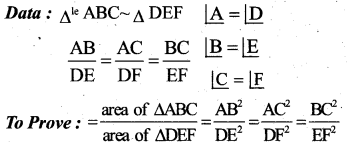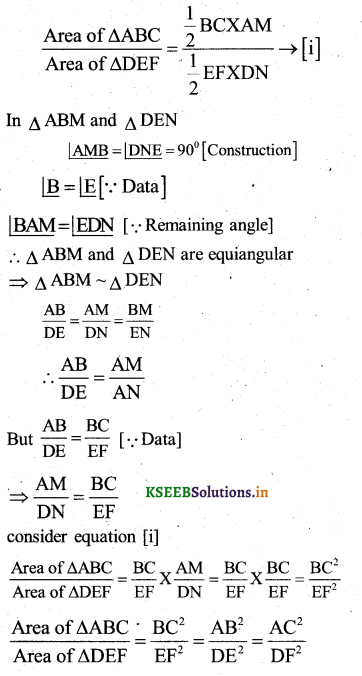# Karnataka SSLC Maths Model Question Paper 3 with Answers

Students can Download Karnataka SSLC Maths Model Question Paper 3 with Answers, Karnataka SSLC Maths Model Question Papers with Answers helps you to revise the complete Karnataka State Board Syllabus and to clear all their doubts, score well in final exams.

## Karnataka State Syllabus SSLC Maths Model Question Paper 3 with Answers

Time: 3 Hours
Max Marks: 80

I. In the following questions, four choices are given for each question, choose and write the correct answer along with its alphabet: ( 1 × 8 = 8 )

Question 1.
The value of ‘P’ for which the pair of linear equations px-y=2 and 6x – 2y = 3 will have infinitely many solutions is :
a) 1
b) -1
c) 3
d) -3
c) 3
Solution :
Px – y = 2 = 0
6x – 2y – 3 = 0
a1x + b1y + c1 = 0
a1 = c b1 = -1 c1 = -2
a2 = 6, b2= c2 = -3Question 2.
A number is divided by 23 given 27 quotient 4 as remainder is
a) 624
b) 625
c) 626
d) 627
b) 625
Solution:
a = bq + r
a = 23 × 27
a = 621 +4
a = 625Question 3.
The value of $$\frac{2 \tan 60}{1+\tan ^{2} 60}$$ is
a) sin60°
b) sin30°
c) cos60°
d) cos45°
a) sin60°
Solution: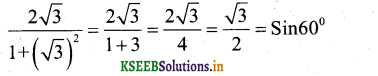Question 4.
The value of x in the co-ordinates of ‘A’ are
a) 3
b) -3
c) 2
d) -2
b) -3
Solution:
$$\frac{x+1}{2}$$ and $$\frac{y+4}{2}$$ = -3
x+4
x = 4- 1
x = 3
y + 4 = -6
y = – 6 – 4
y= -10Question 5
The length of a tangent from a point p at a distance of 5cm from the centre of the circle is 4cm. The diameter of the circle is :
a) 3cm
b) 6cm
c) 8cm
d) 10cm
b) 6cm
Solution :
d2 = r2 + t2
52 = r2 + 42
r2 = 25-16=9
r = 3 cm
d = 2r
= 2 × 3 = 6cm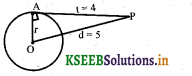Question 6.
The 8th term of an A.P. is 25. The sum of it’s first 15 terms is
a) 310
b) 350
c) 375
d) 400
c) 375
Solution:
S15 = $$\frac{15}{2}$$[2a+14d]
= $$\frac{15}{2}$$ x 2[2a+14d]
=15 × T8
=15 × 25
= 375

Question 7.
A die is thrown once. The probability of getting a prime number is
a) 1
b) 1/2
c) 2/3
d) 1/3
b) 1/2
Solution :
S = {1, 2, 3, 4, 5, 6} n(s)=6 A={2, 3, 5}
P(A) = 3
n(A = $$\frac{n(A)}{n(S)}=\frac{3}{6}=\frac{1}{2}$$

Question 8.
The angle of elevation of a ladder leaning against is 45° and the foot of the ladder is 10m away from the wall. The length of the ladder is
a) 5√2
b) 10√2 c) 5√3 d) 1o√3
b)10√2
Solution :
In ∆ ABC
∠B = 90°∠C = 45° ∠Z = 45°
∴AB = BC = 10
AC2 = AB2 + BC2
ACv = 102 + 102
AC2 = 100 + 100
AC= $$\sqrt{100 \times 2}$$
AC=10√2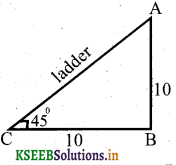II. Answer the following questions: ( 1 x 8 = 8 )

Question 9.
Express 0.2317 in the form of p/q, where p and q are co-prime and also express q in the form 2n x 5m.
0.2317 $$\frac{2317}{10,000}$$ is in the form of p/q P = 2317 and q = 10000 are co-primes
Now
q = (10)4= (2 × 5)4=24 × 54

Question 10.
If one of the roots of the quadratic equation 3x2+ px – 4 = 0 is 4. Find the value of P.
Solution:
x = 4 is one of the roots of the equation.
3x2 + px – 4 = 0
3(4)2 + p(4) – 4=0
48 + 4p – 4 = 0
4p + 44 = 0 ‘
4p = -44
P = -44/4
P = -11

Question 11.
If A+B=90°, Cot B = $$\frac{3}{4}$$ then find the value of SinA
Solution :
In ∆ ABC
∠A + ∠B=90° (given)
.’. ∠C=90°
∠A < 90, ∠B < 90°
$$\frac{\mathrm{adj}}{\mathrm{opp}}=\frac{\mathrm{BC}}{\mathrm{AC}}=\frac{3}{4}$$
AB2 = AC2 + BC2
AB2 = 42 + 32
AB2= 16 + 9
AB2 = 25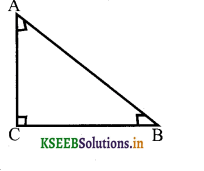AB = √25
AB = 5
∴ Sin A = $$\frac{\mathrm{opp}}{\mathrm{hyp}}=\frac{\mathrm{BC}}{\mathrm{AB}}=\frac{3}{5}$$Question 12.
Find the fourth co – ordinate of the parallelogram ABQD.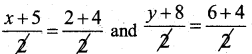x+ 5 = 6
x = 6 – 5
x = 1
y + 8 = 10
y = 10 -8
y = 2
∴ D(x,y) = (1,2)Question 13.
A bag contains 4 .red balls and 3 black balls. A ball is drawn at random. What is the probability of drawing a red ball?
Total number? of balls = 4 + 3 = 7
n(s) = 7
Number of red balls = n(A)=4
A ball is drawn at random
∴ P(A) = $$\frac{\mathrm{n}(\mathrm{A})}{n(s)}=\frac{4}{7}$$

Question 14.
Calculate the perimeter of a circle of area 154cm2?
Area of a circle = 154cm2
πr2 = 154
r2 = 154/π
r2 = 154 x $$\frac{7}{22}$$
r2 = 49
r = 7
C = 2πr = 2 x $$\frac{7}{22}$$ × 7
= 44cm

Question 15.
Write the formula to find the volume of the frustum of a cone.
V= $$\frac{1}{3}$$ π ( R2 +r2 + Rr) h

Question 16.
Find the area of the shaded region in which ABCH a sqaure of each side √98 cm
= πr2 – a2
$$\frac{7}{22}$$ × 7 – 98
= 22 × 72 – 98
= 154 – 98
= 56cm2III. Answer the following: ( 2 × 8 = 16 )

Question 17.
Prove that √2 + √3 is an irrational number.
Let us assume √2 + √3 a Rational number.
⇒ √2 + √3 =a where a is a rational number
√3 = a-√2
Squaring on both sides.
(√3)2 = (a-√2 )2
3 = a2 + ( √2 )2 – 2a √2 ⇒ 3 = a2 + 2 – 2a√2
2√2 = a2 + 2 – 3
√2 = $$\frac{a^{2}-1}{2 a}$$
From this √2 is a rational number because $$\frac{a^{2}-1}{2 a}$$ is rational. But we know is irrational.
This leads to a contradiction. Hence, our assumption that A/2 + A/3 is rational is wrong
Hence √2 + √3 is an irrational number.

Question 18.
Prove that the area of the equilateral traingle described on the side of a square is half the area of the equilatiral triangle described on it’s square.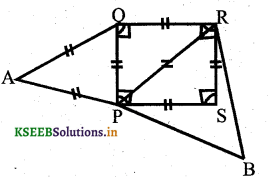PQRS is a sqaure.
∴ PQ = QR = RS = PS = ‘a’ units
PR2 = PS2 + RS2
PR2 = a2 + a2 = 2a2
PR = √2a2
= a√2
∆ PAQ and ∆ BPR are equilateral
∴ ∆ PAQ~∆BPROR

In ∆ ABC D,E,F are the midpoints of the sides BC, AC and AB respectively. Find the ratios of the areas of ∆ DEF ∆ ABC
D.E joins the midpoints of AC & BC
∴ DE||AB,
∴ AFDE, BDEF and CDFE are parallelograms∆ ABC and ∆ DEF
are equiangular.
∴ ∆ ABC~∆ DEF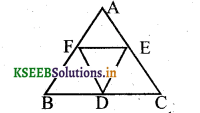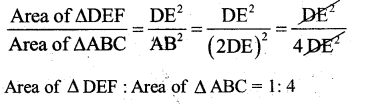Question 19.
Mention the following with respect to the cubic polynomial ax3 + bx2 + cx + d
a) Sum of the zeroes
b) Sum of the product of the zeroes, taken two at a time.
Cubic polynomial is ax3 + bx2 + cx + d where a ≠0
a) Sum of the zeroes-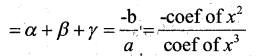b) Sum of the product of the zeroes, taken two at a time. –The Polynomial Roots Calculator will find the roots of any polynomial with just one click. Finding roots of polynomials was never that easy!

Question 20.
Draw a circle of radius 4cm and construct a pair of tangents to the circle which are inclined to each other at an angle of 45°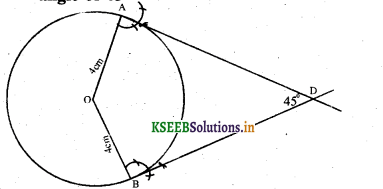Question 21.
If α and β are the zeroes of the polynomial f(x) = 3x2 + 5x + 7, then find the value of $$\frac{1}{\alpha^{2}}+\frac{1}{\beta^{2}}$$
Given : f(x) = 3x2 + 5x + 7
f(x) = ax2 + bx + c a = 3, b = 5, c=7
Let α and β are the roots of f(x)Question 22.
The angle of elevation of the top of an under construction pillar is 100m from its base is 45° calculate the height when the pillar be raised so that its angle of
elevation at that point is60° (Take √3 = 1.73
In ∆ABC
Tan 45° = BC/AB
I = BC/AB
∴ BC = AB = 100cm
In ∆ABD
Tan 60° = BD/AB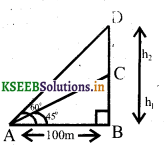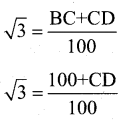100 + CD = 100√3
CD = 100√3 – 100
CD = 100 × 1.73-100
CD =173-100
CD = 73m

OR
A solid metalic right circular cylinder 1.8m high with diameter of its base 2m is melted and recast into a right circular cone with base of diameter 3m. Find the height of the cone.
Cylinder : h1 = 1.8cm, d = 2m ⇒ r =2/2 = 1m
Cone : d = 3m, R= 3/2 =1.5m, h2=?
Volume of cylinder = Volume of cone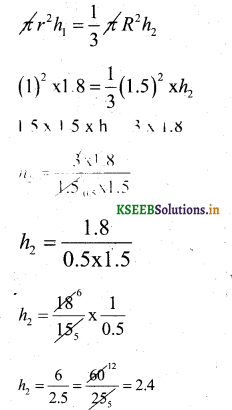h2 = 2.4m
height of the cone = 2.4 m

Question 23.
Find the area of shaded region in the given figure.
OB = $$\sqrt{7^{2}+7^{2}}=\sqrt{49 \times 2}=7 \sqrt{2}$$
= Area of sector – Area of squareQuestion 24.
Find the maximum volume of a cone that can be carved out of a solid hemi¬sphere of radius 21cm.
Solution :
height of cone = radius of hemisphere
∴ Volume of cone = $$\frac{1}{3}$$ πr2h
$$\frac{1}{3}$$ 212 × 21
= 22 x 441
= 882 / 9702
= 9702ccAnswer the following questions ( 8 × 9 = 72 )

Question 25.
2 tables and 3 chairs together cost Rs. 4000 whereas 3 tables and 2 chairs together cost Rs. 5000. find the cost of each table and chair.
Let the cost of each table be Rs x and each chair be Rs.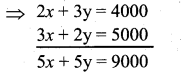Divide by 5
x + y=1800
Subtracting : 1 and 2∴ cost of each chair = Rs. 400
consider, x + y = 1800
x +400 = 1800
x = 1800 + 400
x = 1400 Rs.
Cost of one Table = Rs. 1400/-

OR

The sum of a two digit number and the number formed by interchanging the digits is 110. If 10 is substracted from the first number, the new number formed is 4 more, than 5 times the sum of the digits of the first number. Find the number.
Let the number in the units place of digit number be ‘y’ and in tens place be x.
∴ The number = 10x + y
The new number formed by interchanging
digits = 10y + x
According to the data
(10x+y)+( 10y+x)= 110 ……(1)
=> 11x + 11y = 110 Or x + y=10
and (10x + y)-10 = 5(x + y) + 4
10x + y – 10 = 5x + 5y+4
10x – 5x + y – 5y = 4 +10
5x – 4y = 14 ….. (2)
From (1) and (2)
(x + y = 10 ) × 4Consider x + y = 10
6 + y = 10
y = 10 – 6 = 4
y = 4
∴ The two digit number = 10x + y
= 10(6)+4
= 60 + 4
= 64

Question 26.
The Sum of squares of 3 consecutive natural numbers is 194. Find the numbers.
Let the three consecutive natural numbers be x, x+1, x+2
sum of their squares =194
x2 + (x+1)2 + (x+2)2 = 194
x2 + x2 + 2x + 1 + x2 + 4x + 4 = 194
3x2 + 6x+5- 194 = 0
3X2 + 6x -189 = 0
Divide by 3
x2 + 2x – 63 = 0
x2 + 9x – 7x – 63 = 0
x(x + 9) – 7( x + 9)=0
(x + 9) (x – 7)=0
x + 9 = 0 or x – 7 = 0
x = -9
x = 7 .Question 27.
Find the area of a triangle whose vertices are (-5, -1), (3,-5) and (5,2)
x1 = 5, y1=-1, x2 = 3, y2 =.-5, x3 =5, y3 = 2
Area of ∆le = $$\frac{1}{2}$$ [x1(y2 – y3) + x2(y3 – y1) + x3(y1 – y2)
=$$\frac{1}{2}$$[-5 (-5-2) + 3(2 +1) + 5(-l + 5)] = i[(-5)(-7) + 3(3) + 5(4)]
= $$\frac{1}{2}$$ (+35 + 9 + 20)
= $$\frac{1}{2}$$(+35+29).
= $$\frac{1}{2}$$ x (+64)
= +32
Area of ∆le = 32 sq.units.

OR

The respective vertices of a Rhombus are (3,0), (4,5), (-1,4) and (-2,-1). find the area of the Rhombus.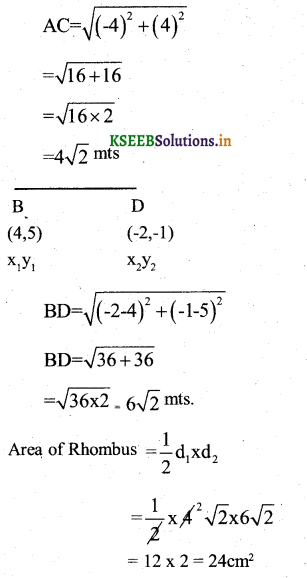Question 28.
Prove that, the tangents drawn from an external point to a circle subtend equal angle at the centre”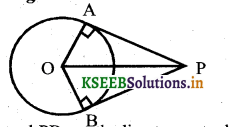Data : PA and PB areBthe line tangents drawn from an external point P to the circle centred O. OA and OB are radius. OP joins the centre and the external point.OP = OP [∵ common]
OA = OB [ ∵ Radii of same circle]
By RHS postulate
∆AOP= ∆ BOP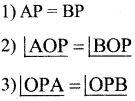Question 29.
A right circular metalic cone of height 2.7cm of radius of its base is 1.6cm. It is melted and recast to form a sphere. Find the radius of the sphere formed.
r = 1.6cm, h1 = 2.7 cm l = ?
Volume of sphere = volume of cylinderOr

A right circular cone is mounted ona cylinder of base radius 14m height of the cylinder is 3m and that of the cone is 10.5m. Calculate the C.S.A. of the solid and cost of paint¬ing at Rs. 20 per sq.m.
l2 = r2 + h2
l2 = 142 + (10.5)2 = 196 + 110.25
1 = 306.25 = 17.5m
C.S.A. ofsolid=C.S.AofCylinder+C.S.A of cone
=2 πrh + πrl
= 88 x 3 + 35 x 22= 264 + 770
= 1034m2
∴ cost of paintmg 1034 x 20
= Rs.20680Question 30.
Draw a Cumulative Frequence curve for the following frequence distribution by less
method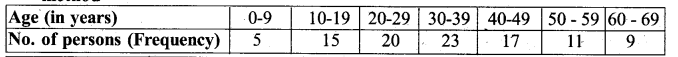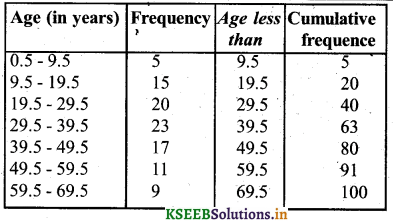Question 31.
Solve the pair of linear equations graphically : 2x – y = 2 and 4x – y = 8
2x – y = 2
4x – y = 8
2x – y = 2
2x = 2 + y
x = $$\frac{2+y}{2}$$4x – y = 8
x = $$\frac{8+y}{4}$$VerifyVerify
2x – y = 2
2(3) – y=2
-y = 2 – 6
-y = – 4
y = 4Question 32.
The sum of the first ‘n’ terms of an A.P is $$\frac{3 n^{2}}{2}+\frac{5 n}{2}$$ Find it’s 50th term
Given Sn = $$\frac{3 n^{2}}{2}+\frac{5 n}{2}$$
We know that T =Sn -Sn-1OR

Find the Sum of Natural numbers be¬tween 200 to 300 which are divisible by 7.
203 + 210 + 217 + 224 + …. + 294
a=203, T =294, n=? S =?, d = 7
Tn=a+(n-1)d
294 = 203 + (n-l)7
n – 1 = $$\frac{294-203}{7}=\frac{91}{7}$$
n-1 = 13
n = 13 + 1
n= 14
sn = $$\frac{n}{2}$$ (a + l )
s14 = $$\frac{14}{2}$$ (203 + 294 )
=7(497)
=3479

Question 33.
A train travels a distance of 480 kins at a uniform speed. If the speed had been 8km/h less, then it would have taken 3 hours more to cover the same distance calculate the speed of the train.
Let the speed of the train be “JC” kmph dis-tance covered = 480 km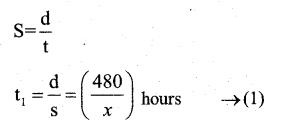The speed is less by 8km/hr then the speed (x-8) kmph and d = 480 km.The difference in time = 3 hours.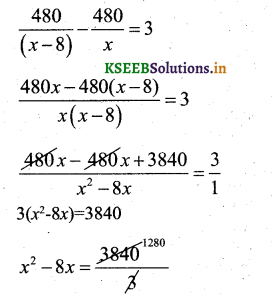x2 – 8x – 1280 = 0
x2 -40x+32x-1280=0
x(x-40)+32(x-40)=0
(x-40) (x+32)=0
x – 40 = 0 OR x + 32 = 0
x = 40
x = -32
∴The average speed of the train is 40km/hr.

OR

The altitude of a right triangle is 7cm – less than its base. If the hypoteneuse is I3cm. find the other two sides.
Let the base be x cm
∴ The altitude = (x-7)cm
Hypoteneuse = 13 cm.
According to pythagorous theorem.
In A ABC, ∠B = 90°
AC2 = AB2 + BC2
132 = (x-7)2+x2
169 = x2 – 2(x)(7)+72+x2
169 = 2x2 – 14x + 49
2x2– 14X+49-169=0
2x2 – 14x – 120=0
Divide by 2
x2 – 1x – 60 = 0
x2 – 12 + 5x – 60 = 0
x(x – 12) + 5(x – 12)=0
(x – 12) (x + 5) = 0
x – 12 = 0 OR x + 5 = 0
x = -5 x = 12
BC = x = 12cm and AB = x-7
12 – 7 = 5cm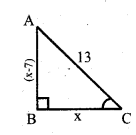Question 34.
The sum. of 4 numbers in AP is 32 and the sum of their squares is 276. Find the numbers.
Let the four numbers in A.P be a – 3d, a – d, a+d, a + 3d
Sum of four terms = a-3d + a-d+a+d+a+3d=32
4a = 32
=> a = 32/4 = 8
Sum Of their squares :
(a – 3d)2 + (a – d)2 + (a+d)2+(a+3d)2 = 276
(8 – 3d)2 + (8 + 3d)2+(8 – d)2 + (8 + d)2 = 276
2[82 + (3d)2] + 2[82 + d2] = 276 2 [64+9d2]+2 [64+d2] = 2 7 6
20d2 + 256 =276
20d2 = 276 – 256 = 20
d2= 20/20 = 1
d2 = 1
d = ±l
The four numbers in A.P are
a-3d, a-d, a+d, a+3d
8-3, 8-1, 8+1, 8+3
5,7,9,11
if d = -1.
A.P. = 11,9,7,5,…..
The numbers are 5, 7, 9, 11

OR

Show that the sum of an A.P. whose first term is ‘a’. Second term is ‘b’ and the last term is ‘c’ is equal to
$$\frac{(a+c)(b+c-2 a)}{2(b-a)}$$
T1= a, T2= b,Tn= c, d = b-a, n = ? Sn = ?
Tn = a + (n  -1)d
C = a + (n – 1) (b  -a) .
(c – a) = (n – 1) (b – a)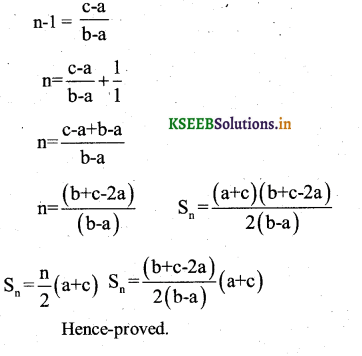Question 35.
Calculate the mean and mode for the following data.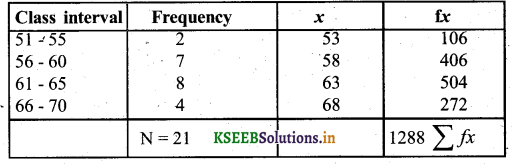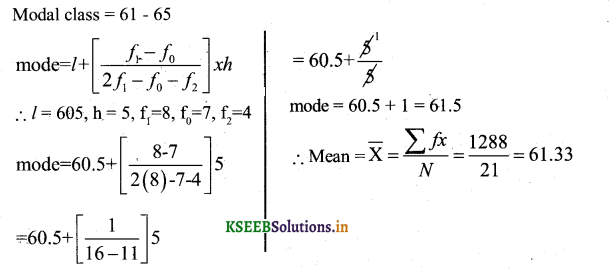Question 36.
Show that $$\frac{\sin \theta}{1+\cos \theta}+\frac{1+\cos \theta}{\sin \theta}=2 \csc \theta$$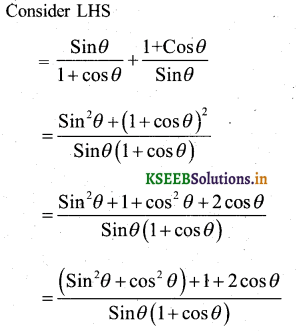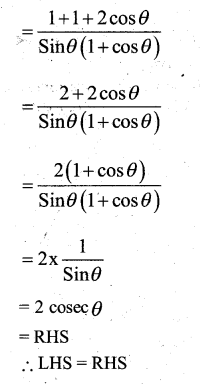Question 37.
Construct a triangle with sides 5cm. 6cm and 7cm and then another triangle whose sides are $$\frac{7}{5}$$ of the corresponding sides of the first triangle.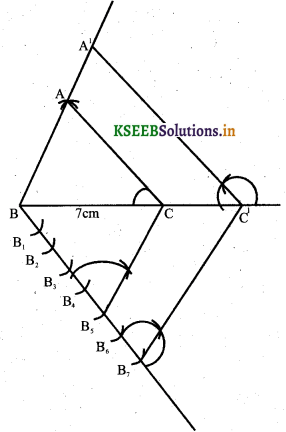VI. Answer the following : ( 5 x 1 = 5 )

Question 38.
Areas of similar triangles are proportional to the squares on the corresponding sides.